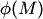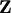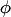# Statistical And On-line Compression Modelling

In standard statistical modelling Reality is modelled as a family probability measures. In on-line compression modelling Reality is modelled as a 5-tuple whose key elements are the forward functions and backward kernels. With each on-line compression modelwe can associate the statistical modeldefined as the extreme points of the probability measures on(whereis the example space) that agree with the on-line compression model. Natural questions (some rather vague) are:

• What are the statistical models that can be obtained in this way (are of the formfor some)?
• Characterize the on-line compression modelsfor which there is no "loss of information" in replacingby.
• Doesestablish a bijection between some wide and natural classes of on-line compression and statistical models?

Some work in this direction has been done by Martin-Lof, Lauritzen, and other authors for repetitive structures (models very closely related to on-line compression models). See Vovk et al. (2005), Section 8.8.

Bibliography